# Area of Trapezoid

Go back to  'Area'

 1 What is a Trapezoid (Trapezium)? 2 Definition of Area of a Trapezoid 3 Formula of Area of a Trapezoid (with Proofs Using Two Illustrations) 4 Important Notes on Area of a Trapezoid 5 Area of a Trapezoid Calculator 6 Solved Examples on Trapezoid 7 Practice Questions on Trapezoid 8 Thinking out of the Box! 9 Maths Olympiad Sample Papers 10 Frequently Asked Questions (FAQs)

We at Cuemath believe that Math is a life skill. Our Math Experts focus on the “Why” behind the “What.” Students can explore from a huge range of interactive worksheets, visuals, simulations, practice tests, and more to understand a concept in depth.

Book a FREE trial class today! and experience Cuemath’s LIVE Online Class with your child.

## What is a Trapezoid (Trapezium)?

A trapezoid (trapezium) is a quadrilateral in which one pair of opposite sides are parallel.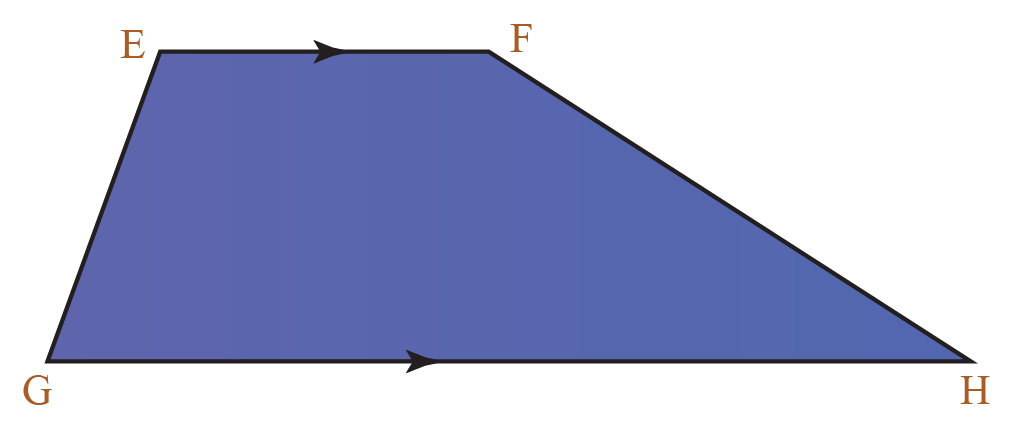• The sides that are parallel to each other are called bases.
In the above figure, $$EF$$ and $$GH$$ are bases.

• The sides that are not parallel to each other are called legs.
In the above figure, $$EG$$ and $$FH$$ are legs.

If the two non-parallel opposite sides are of equal length, it is called an isosceles trapezoid.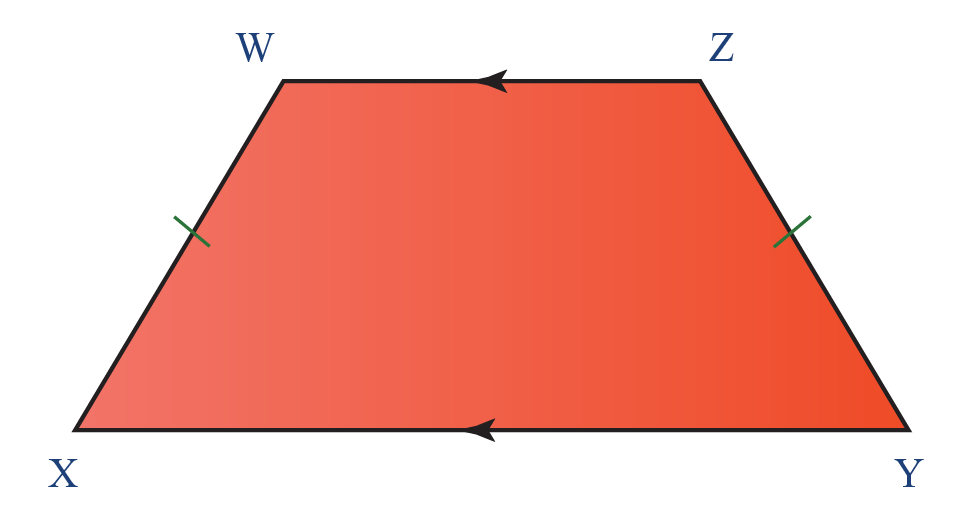The above quadrilateral $$XYZW$$ is an isosceles trapezoid where $$XW=YZ$$

Some real-life examples of a trapezoid are as follows:## Definition of Area of a Trapezoid

The area of a trapezoid is the number of unit squares that can fit into it.

Consider the following trapezoid which is placed on a grid of unit squares.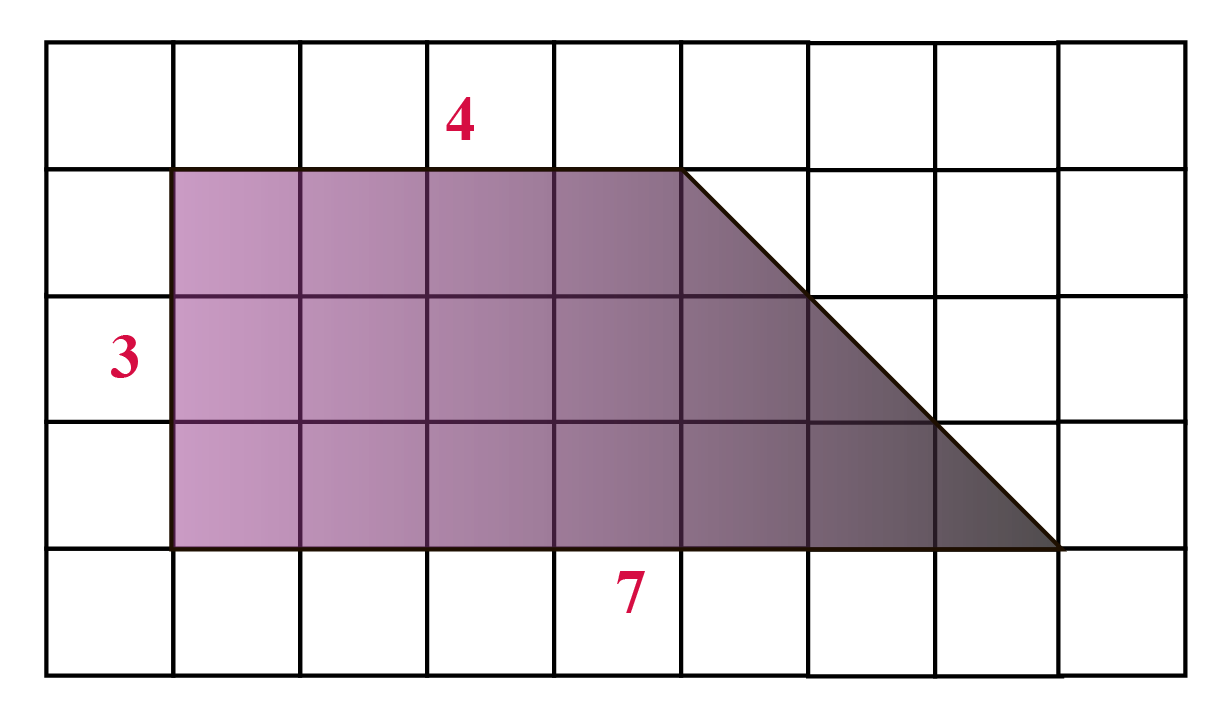Here,

• The number of full unit squares is 15 and the corresponding area is $$15 \times 1=15$$
• The number of half unit squares is 3 and the corresponding area is $$3 \times 0.5=1.5$$

Thus,

\begin{align} \text{The Total Area} &= 15+1.5 \\& =16.5 \text{ square units} \end{align}

 $$\therefore$$ Area of given trapezoid = 16.5 square units

## Formula of Area of a Trapezoid (with Proofs Using Two Illustrations)

Let $$A$$ be the area of a trapezoid whose bases are $$a$$ and $$b$$ and whose height is $$h$$.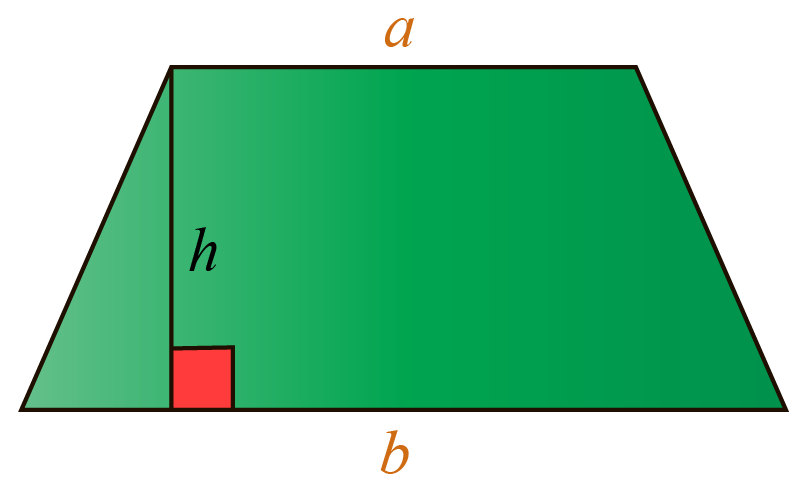$${A=\dfrac{(a+b)h}{2}}$$

It may not always be possible to find the area of a trapezoid by placing it on a grid of unit squares and counting the number of unit squares.

In such cases, we can use the above formula to find the area of the trapezoid.

But how can we derive this formula?

We can derive the formula in two ways.

### Proof by using a Parallelogram

We will consider two identical trapezoids, each with bases $$a$$ and $$b$$ and height $$h$$.

Let us assume that the second trapezoid is turned upside down.

Let $$A$$ be the area of each trapezoid.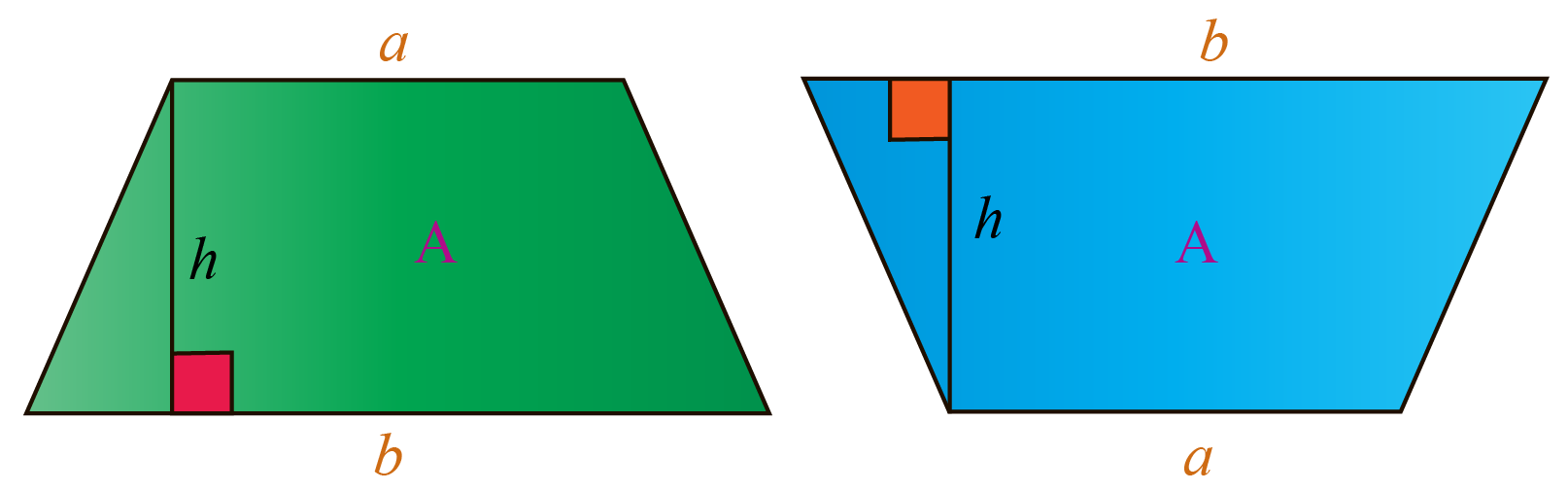Now, we will join the above two trapezoids.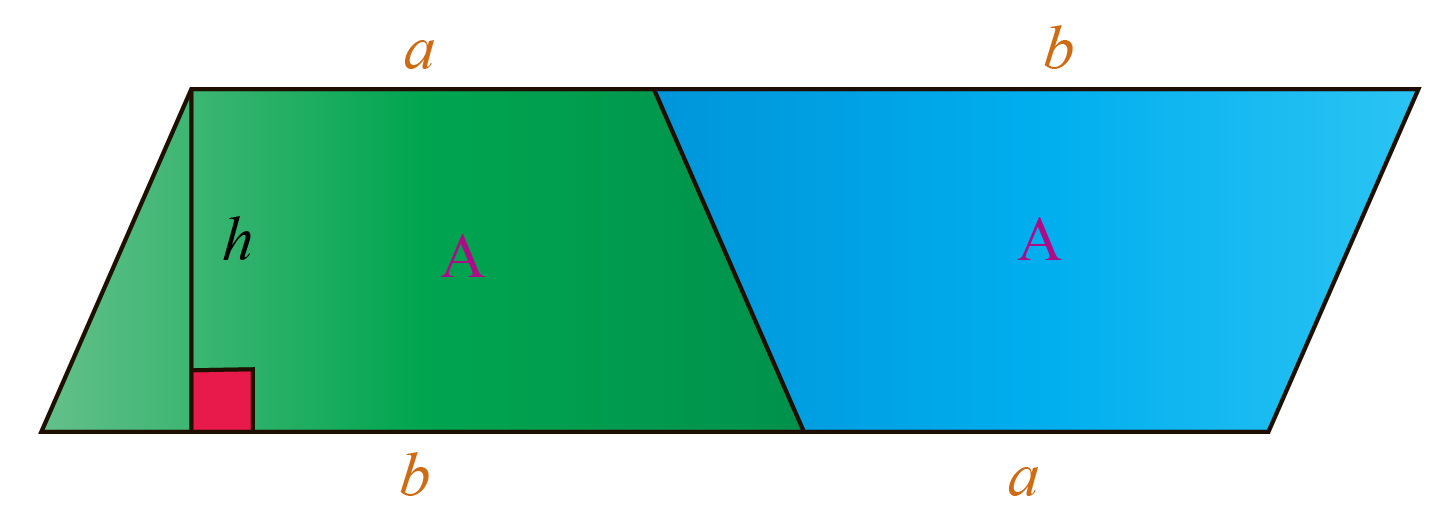We can see that the new figure obtained by joining the two trapezoids is a parallelogram whose base is $$a+b$$ and whose height is $$h$$.

We know that the area of a parallelogram is $$\mathbf{base \times height}$$.

The area of the above parallelogram is, $$A+A=2A$$.

Thus,

\begin{aligned}2A &= (a+b)h \\[0.2cm] A& = \dfrac{(a+b)h}{2} \end{aligned}

Thus, the formula of the area of a trapezoid is proved.

We can understand this proof with the help of the following illustration.

Move the slider slowly to the right to understand the proof.

### Proof by using a Triangle

Let us consider the following trapezoid whose area is $$A$$.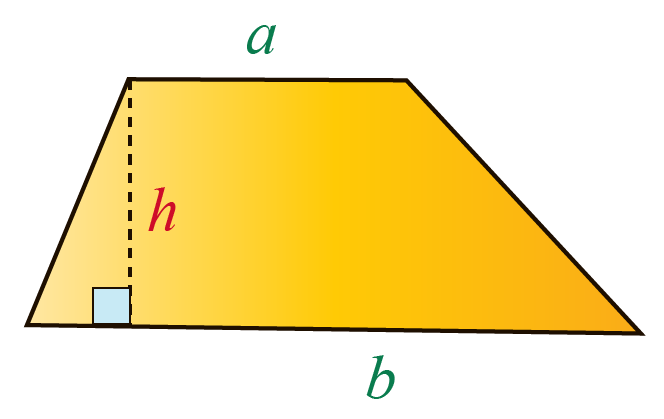We will split one of the legs into two equal parts and cut a triangular portion of the trapezoid.

We will attach this triangular portion at the bottom to get another triangle.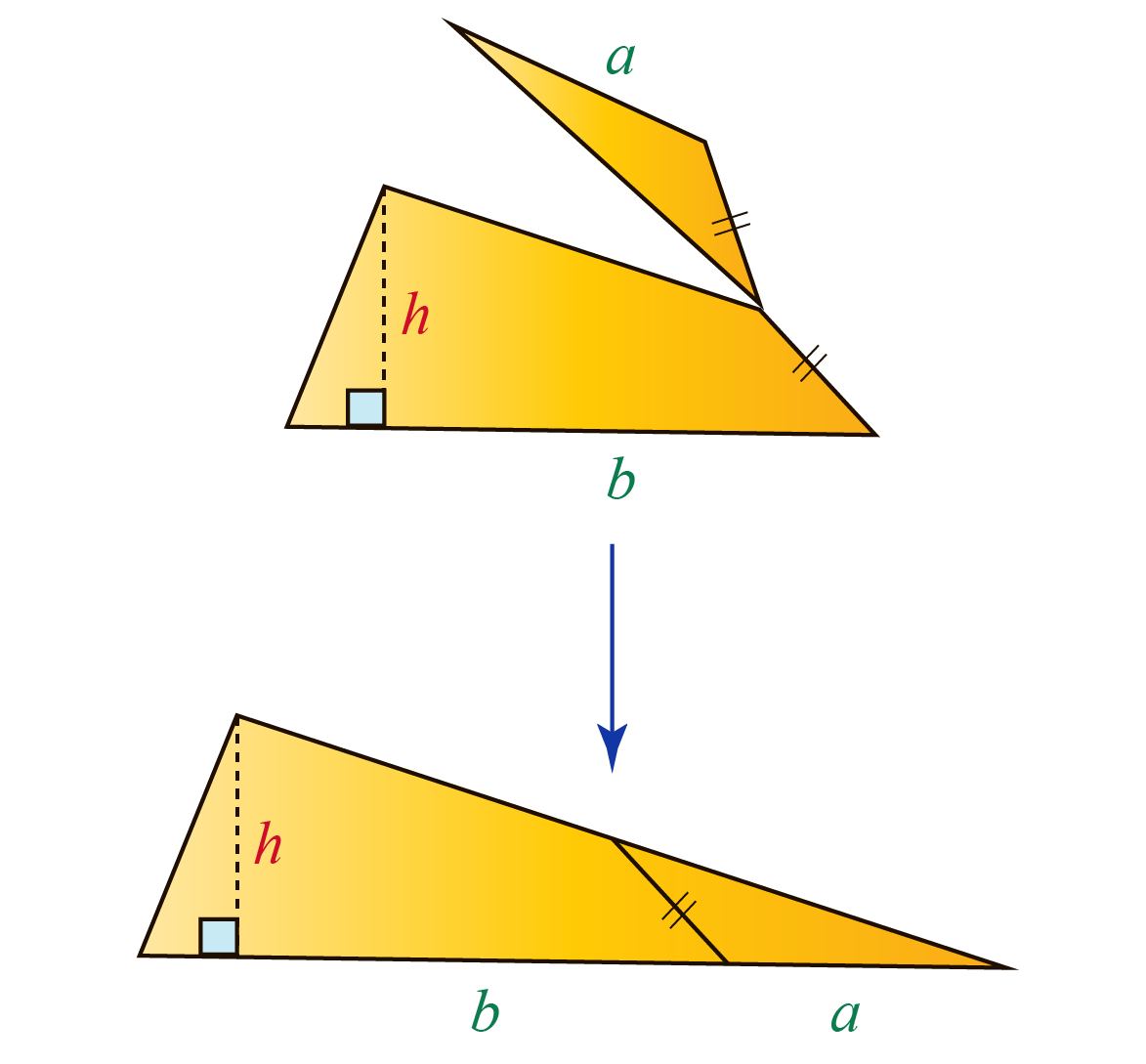Now the trapezoid is converted into a triangle.

The base of the triangle = $$a+b$$ and the height of the triangle = $$h$$.

\begin{aligned} \text{Area of trapezoid }&= \text{Area of triangle}\\[0.2cm] \text{Area of trapezoid }&= \dfrac{1}{2} \times \text{base } \times \text{ height}\\[0.2cm] A&= \dfrac{1}{2}(a+b)h \end{aligned}

Thus, the formula of the area of a trapezoid is proved.

We can understand this proof with the help of the following illustration.

Move the slider slowly to the right to understand the proof.

### Example of Area of a Trapezoid

Here is an example to find the area of a trapezoid.

Find the area of the following trapezoid.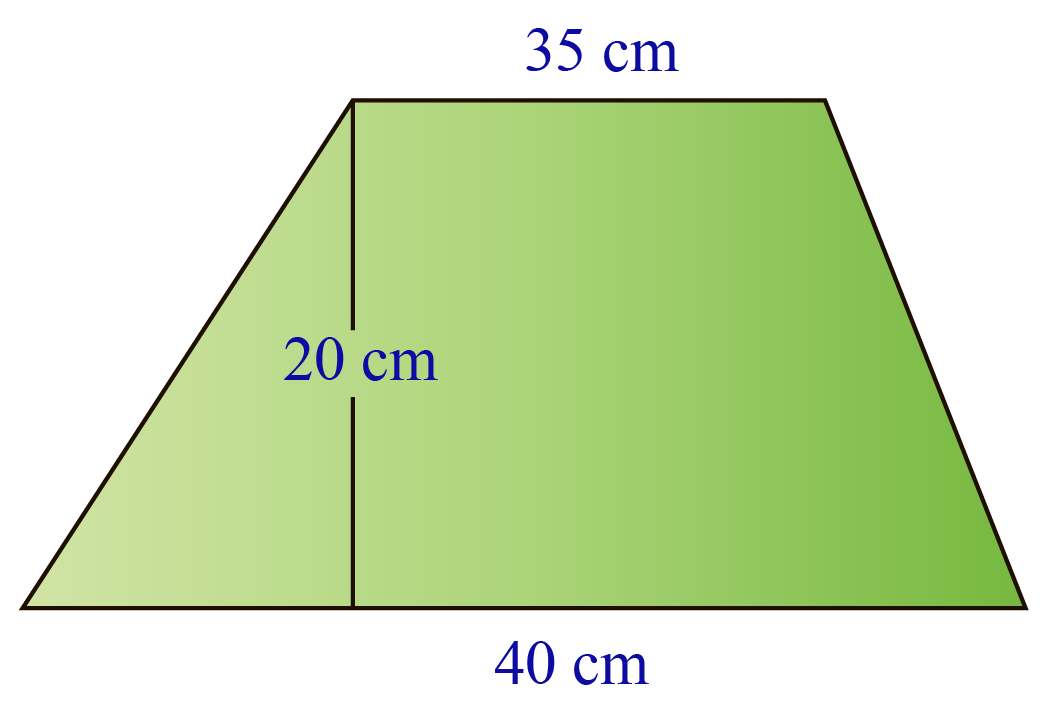We know that the bases are:

$a=35 \text{ cm}\\[0.2cm] b=40\text{ cm}$

The height is:

$h=20\text{ cm}$

The area of the trapezoid is calculated using the formula:

\begin{aligned} A &= \dfrac{(a+b)h}{2}\\[0.2cm] &= \dfrac{(35+40) \, 20}{2}\\[0.2cm] &= \dfrac{(75)\, 20}{2}\\[0.2cm] &= \dfrac{1500}{2}\\[0.2cm] &= 750 \end{aligned}

Thus, the area of the trapezoid is 750 cm2

## Area of a Trapezoid Calculator

The area of a trapezoid calculator is shown below.

We can use it for calculating the area by providing the values of the bases and height of the trapezoid.

Help your child score higher with Cuemath’s proprietary FREE Diagnostic Test. Get access to detailed reports, customised learning plans and a FREE counselling session. Attempt the test now.

## Solved Examples

Here are some examples that show how the area of a trapezoid is calculated.

 Example 1

The area of a trapezium is 128 cm2

If the lengths of the bases are 12 cm and 20 cm, find the distance between the bases.

Solution:

Let us assume that the bases are $$a$$ and $$b$$; and the height of the trapezoid is $$h$$.

Using the given information,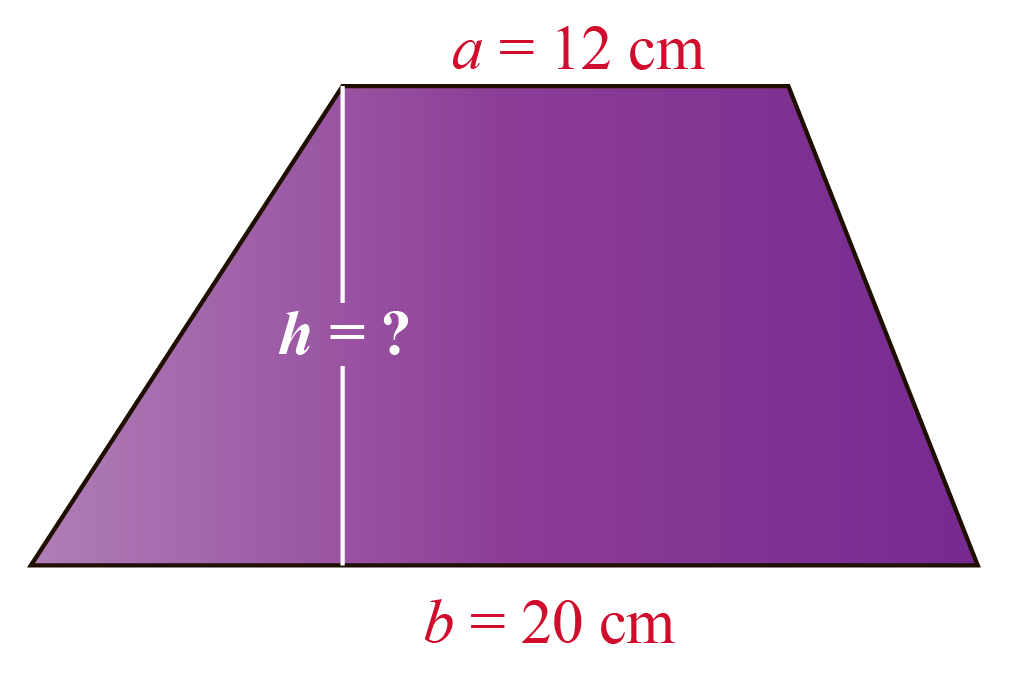We have to find $$h$$ which is the distance or height between the bases.

Let us substitute all these values in the area of a trapezoid formula:

\begin{aligned} A &= \dfrac{(a+b)h}{2}\\[0.2cm] 128&= \dfrac{(12+20)h}{2}\\[0.2cm] 128&= \dfrac{32h}{2}\\[0.2cm] 128&= 16h\\[0.2cm] 8&=h \end{aligned}

Thus,

 $$\therefore$$ Distance between the bases = 8 cm
 Example 2

Find the area of the following trapezoid.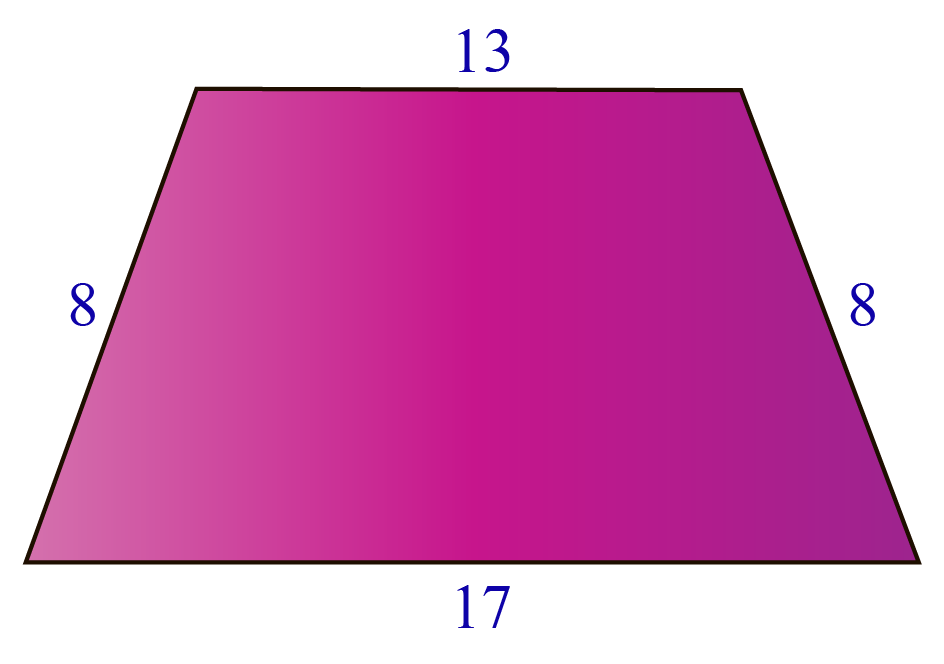Solution:

We will assume that $$a$$ and $$b$$ are the bases, and $$h$$ is the height of the given trapezoid.

The above trapezoid can be represented as follows: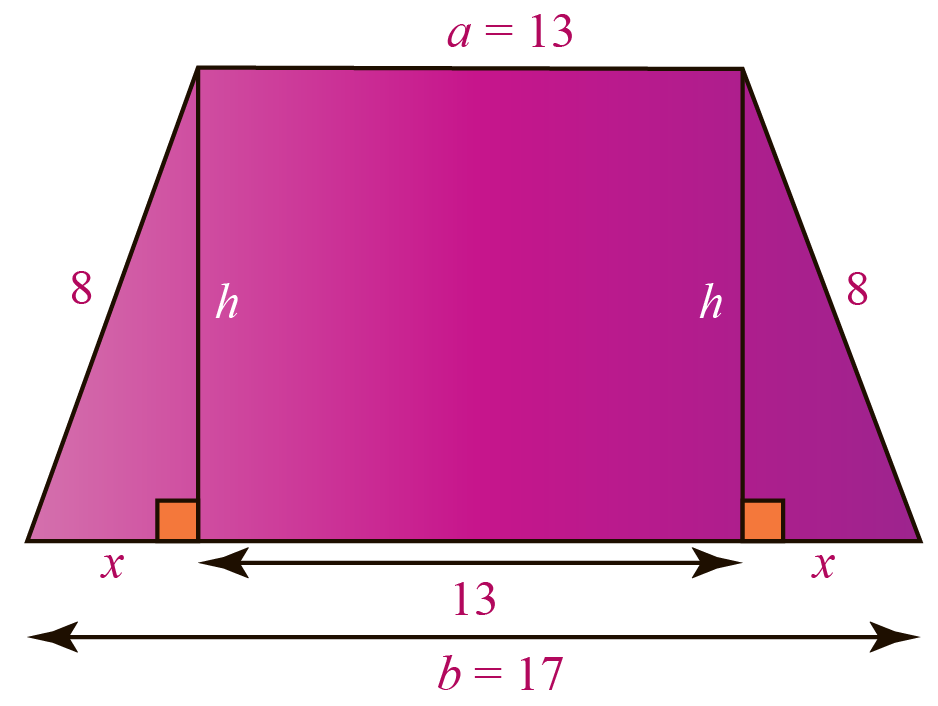From the figure,

\begin{aligned}13+x+x&= 17\\[0.2cm] 13+2x&=17\\[0.2cm] 2x&=4\\[0.2cm] x&=2 \end{aligned}

Now, applying Pythagoras theorem to one of the triangles,

\begin{aligned} 8^2 &= 2^2+h^2 \\[0.2cm] 64&=4+h^2\\[0.2cm] 60&=h^2\\[0.2cm] h &= \sqrt{60}\\[0.2cm] h &= 2 \sqrt{15} \end{aligned}

Now, we will use the area of a trapezoid formula to find its area:

\begin{aligned} A &= \dfrac{(a+b)h}{2}\\[0.2cm] &= \dfrac{(13+17) \, 2 \sqrt{15}}{2}\\[0.2cm] &= \dfrac{(30)\, 2 \sqrt{15}}{2}\\[0.2cm] &= \dfrac{60 \sqrt{15}}{2}\\[0.2cm] &= 30 \sqrt{15} \end{aligned}

Thus, the area of the given trapezoid is:

 $$\therefore$$ A = 30$$\mathbf{\sqrt{15}}$$ units2
 Example 3

Find the area of the front face $$ABCD$$ of the following prism.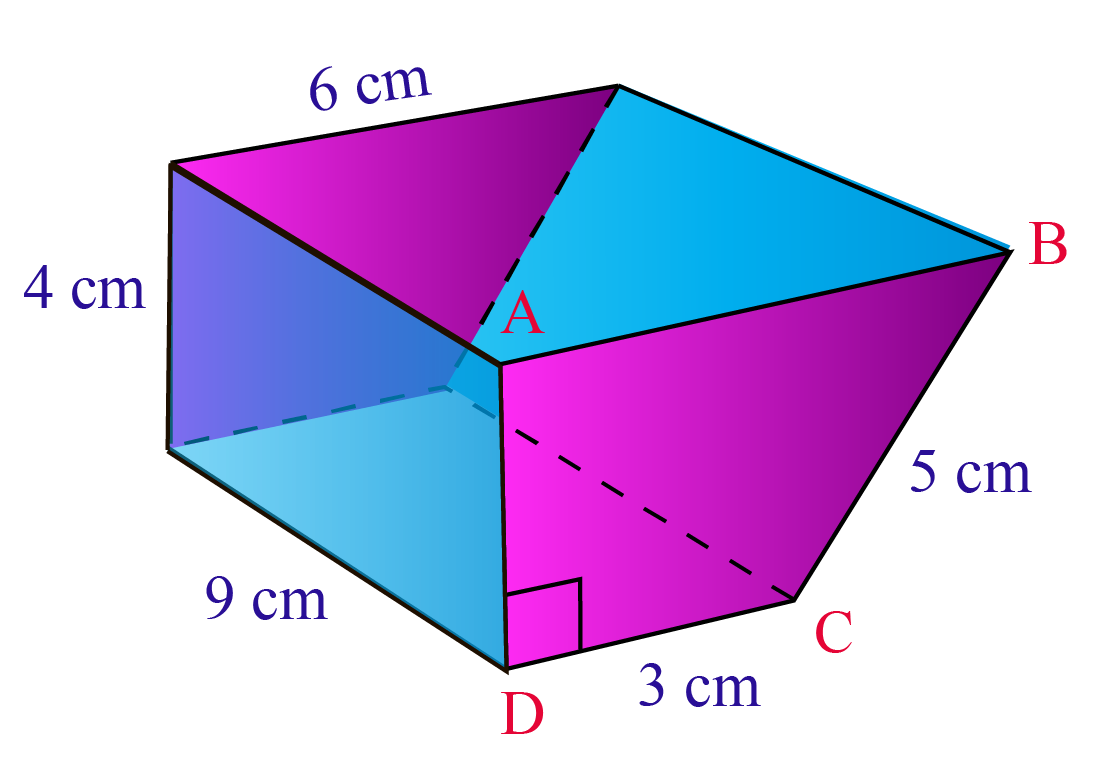Solution:

From the given figure, we can find the length of the bases and the height of the trapezoid.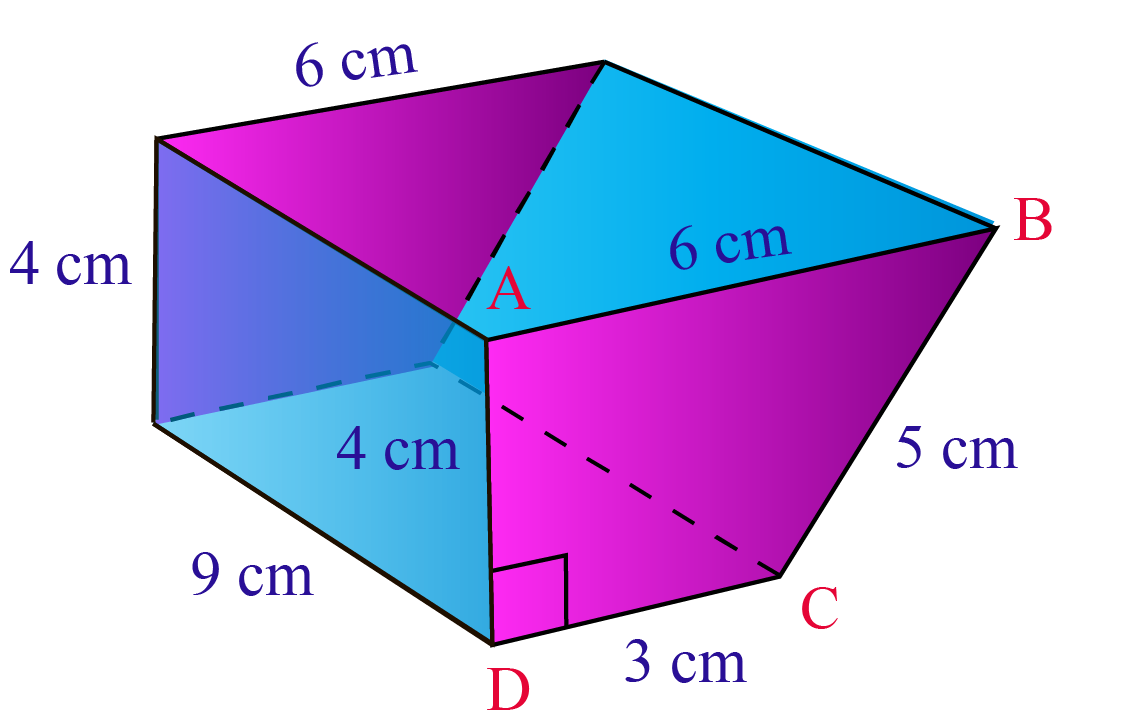Here, the bases of $$ABCD$$ are:

$a=6 \text{ cm}\\[0.2cm] b=3\text{ cm}$

The height of $$ABCD$$ is:

$h=4\text{ cm}$

The area of the trapezoid is calculated using the formula:

\begin{aligned} A &= \dfrac{(a+b)h}{2}\\[0.2cm] &= \dfrac{(6+3) \, 4}{2}\\[0.2cm] &= \dfrac{(9)\, 4}{2}\\[0.2cm] &= \dfrac{36}{2}\\[0.2cm] &= 18 \end{aligned}

Thus, the area of the trapezoid is:

 $$\therefore$$ A = 18 cm2

Want to understand the “Why” behind the “What”? Explore Trapezoid Area with our Math Experts in Cuemath’s LIVE, Personalised and Interactive Online Classes.

Make your kid a Math Expert, Book a FREE trial class today!

## Practice Questions

Here are a few activities for you to practice. Select/Type your answer and click the "Check Answer" button to see the result.Think Tank
1. Find the area of the shaded region in green where $$PQ=40$$ cm, $$RS = 30$$ cm, and the shortest distance between $$PQ$$ and $$RS$$ is $$28$$ cm. Taking $$P, Q, R$$ and $$S$$ as the centers, four arcs of circles of radius $$14$$ cm each are drawn in the following figure.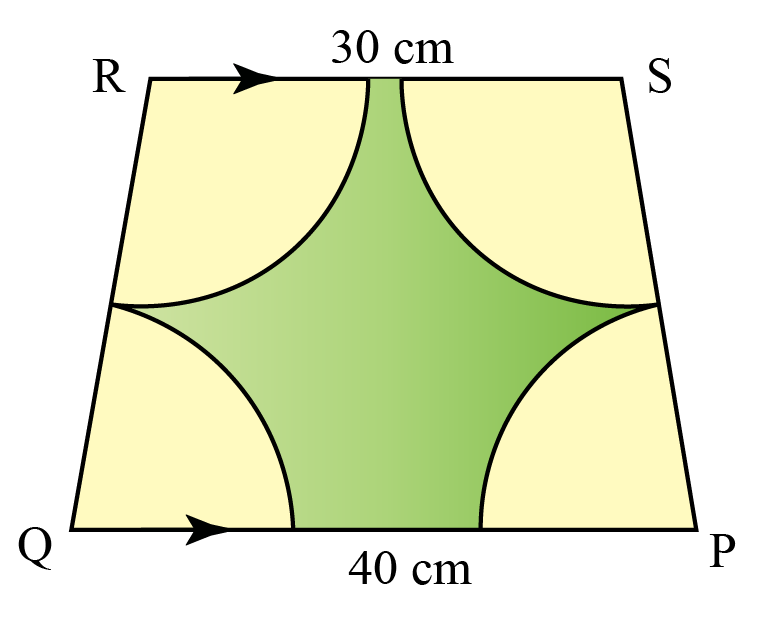IMO (International Maths Olympiad) is a competitive exam in Mathematics conducted annually for school students. It encourages children to develop their math solving skills from a competition perspective.

## 1. How do you find the area of a trapezoid?

The area of a trapezoid whose bases are $$a$$ and $$b$$, and whose height is $$h$$ is calculated using the formula:

$A = \dfrac{(a+b)h}{2}$

The area of a trapezoid formula can be proved in two ways which you can learn under the section "Formula of Area of a Trapezoid".

## 2. What is the formula for the area of a trapezoid?

The area of a trapezoid whose bases are $$a$$ and $$b$$, and whose height is $$h$$ is calculated using the formula:

$A = \dfrac{(a+b)h}{2}$

## 3. How do you use the area of a trapezoid equation?

The area of a trapezoid equation or formula is:

$A = \dfrac{(a+b)h}{2}$

Here, $$a$$ and $$b$$ are the lengths of the parallel sides of the trapezoid, and $$h$$ is the height or the altitude or the distance between the two parallel sides of the trapezoid.

By substituting these values in the above equation, we can find the area of the trapezoid.

More Important Topics
Numbers
Algebra
Geometry
Measurement
Money
Data
Trigonometry
Calculus